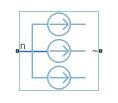# Current Source (Three-Phase)

Ideal three-phase current source

• Library:
• Simscape / Electrical / Sources

•## Description

The Current Source (Three-Phase) block models an ideal three-phase current source that maintains sinusoidal currents of the specified magnitude through its terminals, independent of the voltage across the source.

The output current is defined by the following equations:

`${I}_{0}=\sqrt{2}{i}_{phase_rms}$`
`${i}_{a}={I}_{0}\mathrm{sin}\left(2\pi ft+\phi \right)$`
`${i}_{b}={I}_{0}\mathrm{sin}\left(2\pi ft+\phi -{120}^{\circ }\right)$`
`${i}_{c}={I}_{0}\mathrm{sin}\left(2\pi ft+\phi +{120}^{\circ }\right),$`

where:

• I0 is the peak phase current.

• iphase_rms is the RMS phase current.

• ia, ib, ic are the respective phase currents.

• f is the frequency.

• φ is the phase shift.

• t is the time.

The arrow indicates the positive direction of the current flow. The source has a wye configuration, and port n provides a connection to the center of the wye.

## Ports

### Conserving

expand all

Expandable three-phase port associated with the three phases, a, b, and c.

Electrical conserving port associated with the center of the wye.

## Parameters

expand all

Whether to model composite or expanded three-phase ports.

Composite three-phase ports represent three individual electrical conserving ports with a single block port. You can use composite three-phase ports to build models that correspond to single-line diagrams of three-phase electrical systems.

Expanded three-phase ports represent the individual phases of a three-phase system using three separate electrical conserving ports.

RMS phase current.

Phase shift, in angular units.

Current frequency, specified in Hz or units directly convertible to Hz (where Hz is defined as 1/s). For example, `kHz` and `MHz` are valid units, but `rad/s` is not.

## Version History

Introduced in R2013b